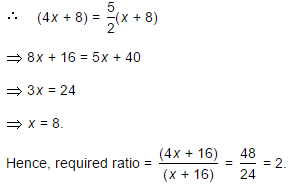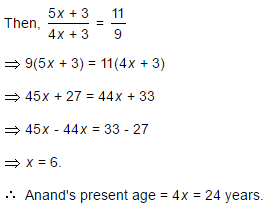CLAT  >  Test: Problems On Ages - 4

# Test: Problems On Ages - 4

Test Description

## 5 Questions MCQ Test Quantitative Techniques for CLAT | Test: Problems On Ages - 4

Test: Problems On Ages - 4 for CLAT 2023 is part of Quantitative Techniques for CLAT preparation. The Test: Problems On Ages - 4 questions and answers have been prepared according to the CLAT exam syllabus.The Test: Problems On Ages - 4 MCQs are made for CLAT 2023 Exam. Find important definitions, questions, notes, meanings, examples, exercises, MCQs and online tests for Test: Problems On Ages - 4 below.
Solutions of Test: Problems On Ages - 4 questions in English are available as part of our Quantitative Techniques for CLAT for CLAT & Test: Problems On Ages - 4 solutions in Hindi for Quantitative Techniques for CLAT course. Download more important topics, notes, lectures and mock test series for CLAT Exam by signing up for free. Attempt Test: Problems On Ages - 4 | 5 questions in 10 minutes | Mock test for CLAT preparation | Free important questions MCQ to study Quantitative Techniques for CLAT for CLAT Exam | Download free PDF with solutions
 1 Crore+ students have signed up on EduRev. Have you?
Test: Problems On Ages - 4 - Question 1

### Father is aged three times more than his son Ronit. After 8 years, he would be two and a half times of Ronit's age. After further 8 years, how many times would he be of Ronit's age?

Detailed Solution for Test: Problems On Ages - 4 - Question 1

Let Ronit's present age be x years. Then, father's present age =(x + 3x) years = 4x years.Test: Problems On Ages - 4 - Question 2

### The sum of ages of 5 children born at the intervals of 3 years each is 50 years. What is the age of the youngest child?

Detailed Solution for Test: Problems On Ages - 4 - Question 2

Let the ages of children be x, (x + 3), (x + 6), (x + 9) and (x + 12) years.

Then, x + (x + 3) + (x + 6) + (x + 9) + (x + 12) = 505x = 20x = 4.Age of the youngest child = x = 4 years.

Test: Problems On Ages - 4 - Question 3

### A father said to his son, "I was as old as you are at the present at the time of your birth". If the father's age is 38 years now, the son's age five years back was:

Detailed Solution for Test: Problems On Ages - 4 - Question 3

Let the son's present age be x years. Then, (38 - x) = x2x = 38.x = 19.Son's age 5 years back (19 - 5) = 14 years.

Test: Problems On Ages - 4 - Question 4

A is two years older than B who is twice as old as C. If the total of the ages of A, B and C be 27, the how old is B?

Detailed Solution for Test: Problems On Ages - 4 - Question 4

Let C's age be x years. Then, B's age = 2x years. A's age = (2x + 2) years.(2x + 2) + 2x + x = 275x = 25x = 5.

Hence, B's age = 2x = 10 years.

Test: Problems On Ages - 4 - Question 5

Present ages of Sameer and Anand are in the ratio of 5 : 4 respectively. Three years hence, the ratio of their ages will become 11 : 9 respectively. What is Anand's present age in years?

Detailed Solution for Test: Problems On Ages - 4 - Question 5

Let the present ages of Sameer and Anand be 5x years and 4x years respectively.## Quantitative Techniques for CLAT

56 videos|35 docs|91 tests
 Use Code STAYHOME200 and get INR 200 additional OFF Use Coupon Code
Information about Test: Problems On Ages - 4 Page
In this test you can find the Exam questions for Test: Problems On Ages - 4 solved & explained in the simplest way possible. Besides giving Questions and answers for Test: Problems On Ages - 4, EduRev gives you an ample number of Online tests for practice

## Quantitative Techniques for CLAT

56 videos|35 docs|91 tests